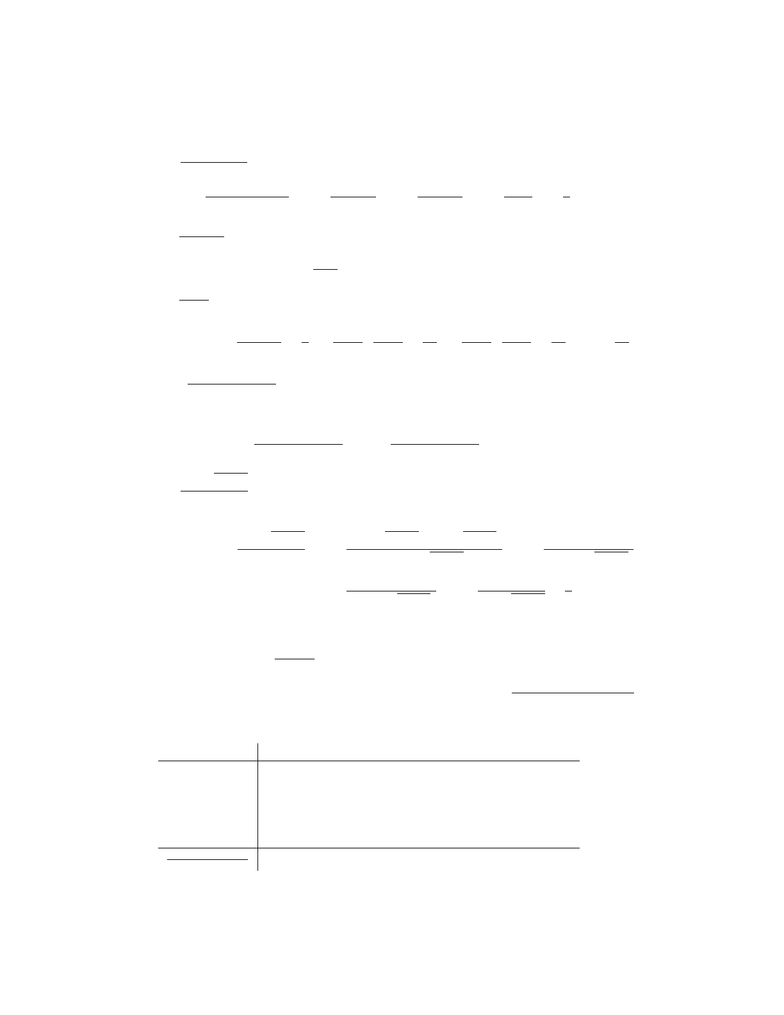# 2006 Test 1 solution

64 views3 pages
School
Department
Course
ProfessorMAT 137Y, 2006–2007 Winter Session, Solutions to Term Test 1
1. Evaluate the following limits. Do not use L’Hˆ
opital’s Rule to evaluate the limit.
(7%) (i) lim
x0
(x1)21
x2+6x
L=lim
x0
x22x+11
x(x+6)=lim
x0
x22x
x(x+6)=lim
x0
x(x2)
x(x+6)=lim
x0
x2
x+6=1
3.
(7%) (ii) lim
t0
sin2(5t)
3t2
Using the property that lim
x0
sinx
x=1 and (as a result of making the substitution x=5t) we have
lim
t0
sin5t
5t=1,
lim
t0
sin2(5t)
3t2=1
3lim
t0
sin5t
t·sin5t
t=25
3lim
t0
sin5t
5t·sin5t
5t=25
3·1·1=25
3.
(7%) (iii) lim
x4+
(4x)|3x14|
|4x|.
If x4+, then x>4, so |4x|=|x4|=x4. Therefore,
lim
x4+
(4x)|3x14|
|4x|=lim
x4+
(4x)|3x14|
x4=lim
x4+−|3x14|=2.
(7%) (iv) lim
x0
39x2
x2.
Multiplying top and bottom by the conjugate, we have
lim
x0
39x2
x2=lim
x0
(39x2)(3+9x2)
x2(3+9x2)=lim
x0
9(9x2)
x2(3+9x2)
=lim
x0
x2
x2(3+9x2)=lim
x0
1
3+9x2=1
6.
2.
(7%) (i) Solve the inequality x23x
Factoring the expression, we are asked to solve the inequality x(x3)
(x2+1)(x+1)(x1)0. The
expression is zero at x=0,3 and undeﬁned at x=±1. To ﬁnd where the expression is positive
or negative, we analyze the sign of each of the factors using a number chart:
x<11<x<0 0 <x<1 1 <x<3x>3
x− − + + +
x3 +
x2+1+ + + + +
x+1+ + + +
x1− − + +
x(x3)
(x2+1)(x+1)(x1)+++
So the inequality holds for x(1,0](1,3].
1
Unlock document

This preview shows page 1 of the document.
Unlock all 3 pages and 3 million more documents.